# Multiplication with Large Numbers

Rachel Mcconnell, Yuanxin (Amy) Yang Alcocer
• Author
Rachel Mcconnell

Rachel is a certified math teacher for grades 6-8. She graduated from the University of Kansas with a bachelors in Middle Math Education. She has taught middle school math for four years throughout Connecticut, Georgia and Italy. She has passed Praxis tests in math, curriculum, and special education.

• Instructor
Yuanxin (Amy) Yang Alcocer

Amy has a master's degree in secondary education and has been teaching math for over 9 years. Amy has worked with students at all levels from those with special needs to those that are gifted.

Learn how to multiply big numbers. Discover multiplication examples, practice problems, walkthroughs, and solutions for multiplying large numbers. Updated: 01/12/2022

Show

## How to Multiply Big Numbers

Multiplication is one of the four basic math operations, along with addition, subtraction, and division. Multiplication is an operation that uses repeated addition. The problem {eq}4 \cdot 3 {/eq} is equal to {eq}4+4+4 {/eq}. The first number is the number in the group and the second number is the number of groups. Most people have the multiplication table of 0-12 memorized, but what if the numbers are greater than {eq}12 \cdot 12 {/eq}? Long Multiplication is the method of multiplying larger numbers. This could be two by two digit numbers, or greater than ten by ten digit numbers! The good thing about long multiplication is that once two by two digit multiplication is understood, there are no bounds! The same exact process is used for larger numbers, the process is simply repeated. Let's look at how to multiply big numbers by outlining it into steps.

### How to Perform Long Multiplication

1. The Set Up: Start by setting up the multiplication problem vertically by setting one number on top of the other. Typically, if the two numbers have different digit amounts, the number with more digits will be on top. This step is important to give the problem organization. Unlike addition problems, the place values do not have to be lined up but do include the decimal point if it is used in the original problem. Use the problem {eq}8330 \cdot 196 {/eq} as an example.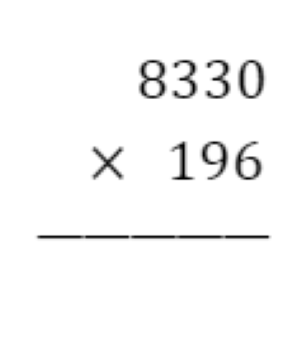2. How Does it Work? Multiplying large numbers together is performed by multiplying the ones digit of the bottom number to the entire top number. After the ones place value has finished multiplying, move to the tens place value in the bottom number and multiply that digit by the top number. But, since this digit is in the tens place, insert a zero place holder into the ones spot in the second line of the solution. This will shift the values to the left one place value, causing the correct multiplication since the number multiplied is not in the ones place value anymore. This might sound confusing in words but there will be plenty of examples below to understand this process.

Look at the progress of the example, the number in the ones place value on the bottom number, 6, has been multiplied by the number 8330. First, {eq}6 \cdot 0 = 0 {/eq}, continue to multiply to the following numbers. {eq}6 \cdot 3 = 18 {/eq}, the 8 goes next to the 0 in the solution but the 1 is placed on top of the 3 in the hundreds place in 8330. This number will be added to the next product. Next multiplication is {eq}6 \cdot 3 = 18 {/eq} but the one needs to be added. Therefore, {eq}18+1= 19 {/eq}. The 9 goes in the solution and the 1 is placed above the 8. Last multiplication, {eq}6 \cdot 8 = 48 {/eq} but add the 1. {eq}48+1= 49 {/eq}. Since this is the last number the 49 can go in the solution because the 4 would be added to 0 which is still 4. Keep in mind, if the product of the multiplication is larger than 9, the tens place of that product must be carried over to the next column and be added to that product.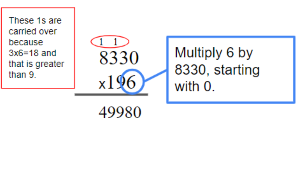3. Repeat: Repeat the process of multiplying one digit in the bottom number by the entire top number and eventually there will be as many rows as there are digits in the bottom number. In this example, there are two more rows to complete. Multiply 9 by 8330, but first put a zero in the ones place. Last, multiply 1 by 8330 but since 1 is in the hundreds place, place two zeros in the solution so the multiplication product is starting in the correct place of the hundreds. View the example below, showing all the completed multiplication.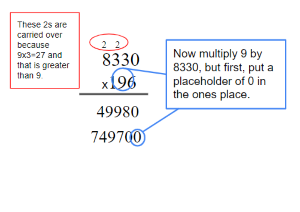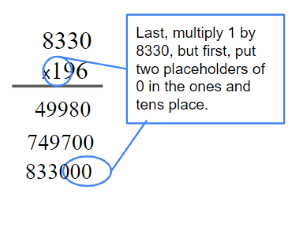4. Add: Remember, multiplication is closely related to addition. In this last step, add the rows together of solutions found by multiplying. After all rows are added, the product has been found!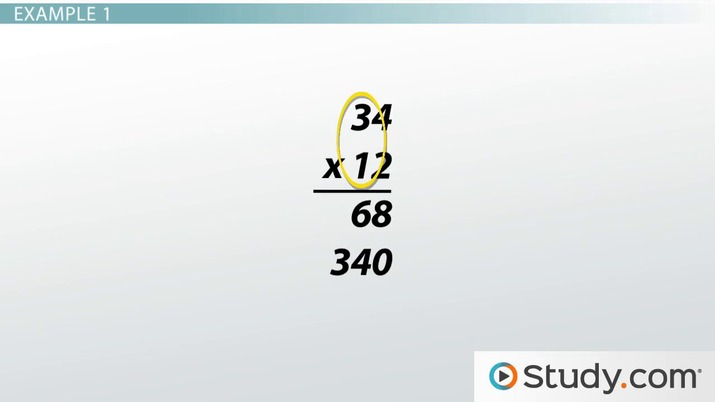An error occurred trying to load this video.

Try refreshing the page, or contact customer support.

Coming up next: How to Perform Division: Steps & Examples

### You're on a roll. Keep up the good work!

Replay
Your next lesson will play in 10 seconds
• 0:01 Multiplying Large Numbers
• 0:58 The Procedure
• 1:48 Example 1
• 4:14 Example 2
• 6:29 Lesson Summary
Save Save

Want to watch this again later?

Timeline
Autoplay
Autoplay
Speed Speed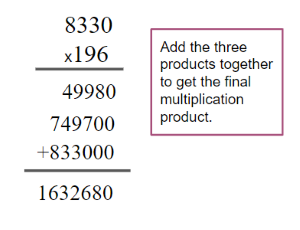## Multiplication Examples

Example One
Multiply the following: {eq}7165 \cdot 42 {/eq}
Solution

1. First set up the problem. Place 7165 on top of 42.

2. Start multiplying 2 by 7165. Below are the multiplication products.

• {eq}2 \cdot 5 = 10 {/eq}, carry the 1 to the next column.
• {eq}2 \cdot 6 = 12 {/eq}, add {eq}12+1=13 {/eq} make sure to place the 1 on top of the next column.
• {eq}2 \cdot 1 = 2 {/eq}, now add {eq}2+1=3 {/eq}.
• {eq}2 \cdot 7 = 14 {/eq}, place the 14 below because there are no more numbers to multiply by the 2.

3. Continue to multiply for the next number, remember to place a zero in the ones place because the first product should go into the tens place value since the 4 is in the tens place value.

• {eq}4 \cdot 5 = 20 {/eq}. Place the 2 in the next column to add to the next product.
• {eq}4 \cdot 6= 24 {/eq}, now add {eq}24+2=26 {/eq} and place the 2 from 26 in the next column.
• {eq}4 \cdot 1 = 4 {/eq}, then add 2 to the product, {eq}4+2=6 {/eq}.
• {eq}4 \cdot 7= 28 {/eq} and place 28 below because there is no more numbers to multiply to 4.

4. Add the rows together. The final answer is 300,930. Check work with the problem below.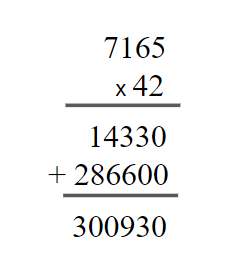Example Two
Multiply the following: {eq}6,533 \cdot 271 {/eq}
Solution

1. First set up the problem. Place 6,533 on top of 271.

2. Start multiplying 1 by 6,533. Below are the multiplication products.

• {eq}1 \cdot 3= 3 {/eq}
• {eq}1 \cdot 3 = 3 {/eq}
• {eq}1 \cdot 5 = 5 {/eq}
• {eq}1 \cdot 6 = 6 {/eq}

3. Continue to multiply for the next numbers, remember to place a zero as a placeholder.

• {eq}7 \cdot 3 = 21 {/eq}, place 2 on top of the next column.
• {eq}7 \cdot 3= 21 {/eq}, add 2 from the previous product, {eq}21+2=23 {/eq} remember to place the 2 on top of the next column to add to the next product.
• {eq}7 \cdot 5 = 35 {/eq}, add 2 to the product, {eq}35+2=37 {/eq} and place 3 on top of the next column.
• {eq}7 \cdot 6 =42 {/eq}, add 3 to the product, {eq}42+3=45 {/eq}.

Now multiply 2 by 6,533. Make sure to put two zeros as placeholders since 2 is in the hundreds place.

• {eq}2 \cdot 3 = 6 {/eq}
• {eq}2 \cdot 3= 6 {/eq}
• {eq}2 \cdot 5 = 10 {/eq} place 1 on top of the next column.
• {eq}2 \cdot 6 =12 {/eq}, add 1 to the product, {eq}12+1=13 {/eq}

4. Add the rows together. The final answer is 1,770,443. Check work with the problem below.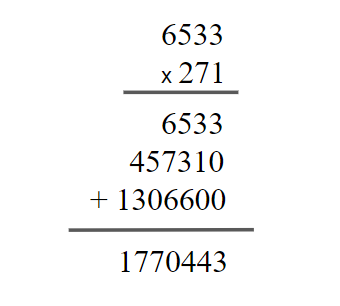Example Three
Multiply the following: {eq}1,883 \cdot 9,200 {/eq}
Solution

1. First set up the problem. Place 9,200 on top of 1,883.

2. Start multiplying 3 by 9,200. Below are the multiplication products.

• {eq}3 \cdot 0= 0 {/eq}
• {eq}3 \cdot 0 = 0 {/eq}

To unlock this lesson you must be a Study.com Member.

#### What is the easiest way to multiply big numbers?

To multiply big numbers, make sure to have a strong base of multiplication tables from 0-9. Use the multiplication products from 0-9 to multiply larger numbers.

#### How do you multiply large numbers?

To multiply large numbers, start by multiplying the ones place of one number by the other number. Follow by multiplying the tens, then thousands, and so on, by the other number but place a zero as a placeholder to move the product to the correct place value. Once the products are found, add the products together to get the final product.

### Register to view this lesson

Are you a student or a teacher?